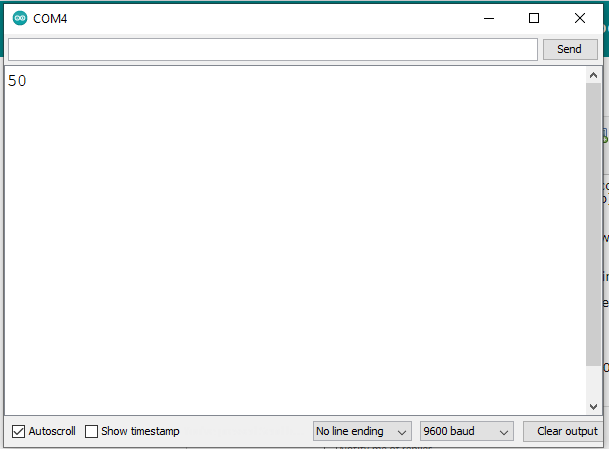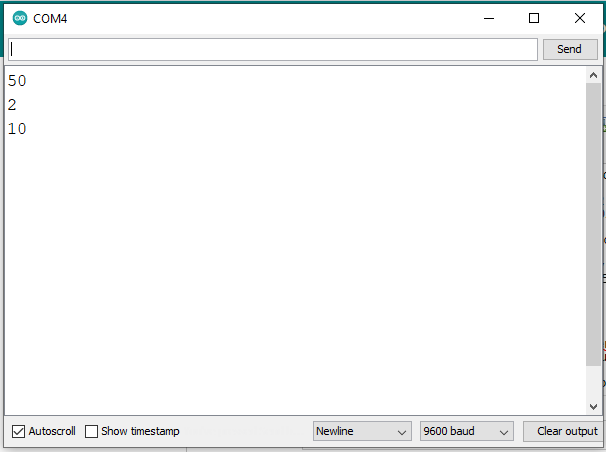# Receiving "random" numbers from Serial.read()

Hello,
I want to send a number from my PC via USB to the arduino and then my arduino to return the same number, so I coded this:

``````int value;
void setup() {
// put your setup code here, to run once:
Serial.begin(9600);
}

void loop() {
// put your main code here, to run repeatedly:
if(Serial.available()){
Serial.println(value);
}

}
``````

But it unfortunately doesn't work, so I tested the example code from arduino.cc:

``````int incomingByte = 0; // for incoming serial data

void setup() {
Serial.begin(9600); // opens serial port, sets data rate to 9600 bps
}

void loop() {
// send data only when you receive data:
if (Serial.available() > 0) {

// say what you got:
Serial.println(incomingByte, DEC);
}
}
``````

but in both cases I'm getting random numbers. Using the example code, I'm getting e.g.:

for 1

I switched the arduino, the usb cable and the only way it works in both cases is writing:

``````Serial.println(char(value));
``````

...or defining the variable as an char.
But I don't understand why this works because Serial.print should be able to handle with integers.
I hope you'll can help me.

Checking an ASCII conversion chart...

That would be the character "1".

Checking the chart again ... that would be CR / carriage return.

Ditto ... that would be LF / line feed.

So, you sent a string. The string consists of the character "1" followed by a carriage return then a line feed.

You need to collect those characters into a string then convert the string to a number.

Okay,
but then the official information on arduino.cc is wrong, right?
It's said that the return of Serial.read() is integer and that Serial.print(value) should output the value (as an int) and doesn't have to be converted from int into char.
Or do I missunderstand something?

LimoCitron:
Okay,
but then the official information on arduino.cc is wrong, right?
It's said that the return of Serial.read() is integer and that Serial.print(value) should output the value (as an int) and doesn't have to be converted from int into char.
Or do I missunderstand something?

You're misunderstanding what the values mean.

Coding Badly already pointed you to the ASCII chart. When you type a 1 into Serial Monitor, you're not sending the number one. You're sending the text character 1, which is represented by number 49. It's also followed by the carriage return and line feed characters which signify a line break (basically hitting ENTER).

If you were to type in a multi-digit number, you wouldn't get a bigger response back, you'd get more responses back, 1 for each digit. 42, for example, would give you back the numbers 52 ("4"), 50 ("2"), 13 ("CR"), 10 ("LF").

If you want to convert the textual information into a number, you need to keep track of all the bytes you receive before the line break, then interpret them into the number they represent.

Serial data arrives as a single byte, that's 8 bits. Not included in that byte is any information about what that byte represents, your code has to supply that. So if you type '1' in the serial monitor then the ASCII character for 1 is sent, which is 49. The code receiving that doesn't know whether 49 represents the number 49, the character 1 or something else you designed, you have to write code to interpret it the way you want. Your code is doing as expected.

Try casting the data you receive to char, that tells the code that you want to interpret the byte as a character.

If you want to interpret a series of arriving characters as a number, there is a function for that:

``````  int integerValue = Serial.parseInt();
float floatValue = Serial.parseFloat();
``````

There is a timeout of (default) one second so if you don't receive any digits in that time the value 0 (or 0.0) will be returned. You can use "if (Serial.available())" to see if there are any characters in the buffer. You can use "while (Serial.available()) Serial.read();" to clear out any characters that may have been left in the buffer, like line-ending characters.

You can choose what line endings the serial monitor sends with the line endings drop down box in serial monitor.

This code will do what you want

``````// readStringUntil_nonBlocking.ino
// Reads chars into a String until newline '\n'
// https://www.forward.com.au/pfod/ArduinoProgramming/SoftwareSolutions/index.html
// Pros: Simple. Non-Blocking
// Cons: Does not return until the until_c char is found. i.e. no timeout or length limit

String input;

void setup() {
Serial.begin(9600);
for (int i = 10; i > 0; i--) {
Serial.print(' '); Serial.print(i);
delay(500);
}
Serial.println();
input.reserve(80); // expected line size
}

// read Serial until until_c char found, returns true when found else false
// non-blocking, until_c is returned as last char in String, updates input String with chars read
bool readStringUntil(String& input, char until_c) {
while (Serial.available()) {
input += c;
if (c == until_c) {
return true;
}
}
return false;
}

void loop() {
Serial.print(F(" got a line of input '")); Serial.print(input); Serial.println("'");
input.trim(); // remove newlines etc
long number = input.toInt();
if (input == String(number)) {
// ok it is a valid number
} else {
Serial.print(input); Serial.print(F(" is not a valid int");
}
input = ""; // clear after processing for next line
}
}
``````

My SafeString library has a fancy SafeStringReader that does more, but the above basically works

@OP

1. This is the layout (Fig-1) of the Serial Monitor.

Figure-1:

2. This is the ASCII Code Table (Fig-2).

Figure-2:

3. This is the sketch that you have uploaded in Arduino UNO.

``````int value;

void setup()
{
Serial.begin(9600);
}

void loop()
{
if (Serial.available())
{
Serial.println(value);
}
}
``````

4. Choose 'No line ending" option for the "Line ending tab" of Serial Monitor (SM, Fig-1).
5. Enter 2 in the InputBox of SM and click on the Send button.
6. What do you you expect to see on the OutputBox of SM? 50 or random numbers?
(1) In response to the action of Step-5, the code 32 (ASCII Code of 2 in hex base, Fig-2) which is 8-bit (00110010) has arrived to the UNO and been stored in Serial Buffer.

(2) You have executed the following code to bring 32 (00110010) from Serial Buffer and keep it into integer type (16-bit) variable value. As you have declared value as a global variable, it has been initialized to 0x0000.

``````value = Serial.read();
``````

(3) 32 (00110011) will occupy the lower 8-bit of value and the upper 8-bit of value variable will remain as 0s(00000000). (For the data source of upper 8-bit, see Note at the end of post.)

(4) You have executed the following line to show the content of the variable value onto the OutputBox of SM.

``````Serial.println(value); //default base is DECimal (10); so, 32 (hex base) will be shown as decimal 50
==> Serial.println(value, DEC);
``````

(5) The OutputBox of SM shows: 50 in SM (Fig-3).
value = 0032 in hex
==> value = 3x161 + 2x160 = 48 + 2 = 50 in decimal.Figure-3:

(6) To see the character (that was entered in the InputBox) back on the OutputBox, the following code could be added in the sketch. The SM now shows (50) 2 (Fig-4).

``````Serial.println((char)value);  //print the character for the ASCII value of 0x32 (50)
``````Figure-4:

(7) The Serial.println(value) command is actually composed two subcommands:

``````Serial.println(value);
==> Serial.print(value);   //ASCII code 0x32 (0x means hex base) travels towards SM
==> Serial.println();          //ASCII code 0x0A (10) for Newline (non-printable char) goes to SM
``````

The Serial.println() command brings the cursor at the next line (also called Line Feed = LF) position.

`** **8)** **`
To verify that Serial.println() really sends ASCII code 0x0A (10), choose Newline option for the Line ending tab of SM and then goto Step-4(5). We will observe the following output (Fig-5) on SM.Figure-5:

Note: The meaning of: int value = Serial.read() returns 16-bit value.
(1) Lower 8-bit of the variable value comes from the Serial Buffer (the ASCII code of 2 in this example).
(2) The upper 8-bit of the value variable comes from UCSR0A Register of MCU. It might have value other than 0 if there happens to be some errors in the received data byte that is coming from Serial Monitor. The errors could be framing error, data overrun etc.This topic was automatically closed 120 days after the last reply. New replies are no longer allowed.